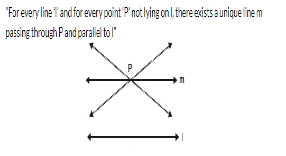# Test: Introduction To Euclid's Geometry - 2

## 25 Questions MCQ Test NCERT Mathematics for CAT Preparation | Test: Introduction To Euclid's Geometry - 2

Description
Attempt Test: Introduction To Euclid's Geometry - 2 | 25 questions in 25 minutes | Mock test for Class 9 preparation | Free important questions MCQ to study NCERT Mathematics for CAT Preparation for Class 9 Exam | Download free PDF with solutions
QUESTION: 1

Solution:
QUESTION: 2

Solution:
QUESTION: 3

### Euclid's Postulate 1 is :

Solution:
QUESTION: 4

Two distinct lines :

Solution:
QUESTION: 5

A line segment when extended indefinitely in one direction is called .................

Solution:
QUESTION: 6

Two distinct points in a plane determine .................

Solution:
QUESTION: 7

For every line l and for every point P not lying on it there exist a unique line which passes through P and is
................. to l.

Solution:QUESTION: 8

If a straight line falling in two straight line make the interior angles on the same side of it taken together, then two straight lines if produced indefinitely, meet on that side on which the sum of angles are ................. 2 right angles.

Solution: If a straight line falling on two straight lines makes the interior angles on the same side of it, taken together less than two right angles, then the the two straight lines if produced indefinitely, meet on that side on which the sum of angles is taken together less than two right angles.
QUESTION: 9

Which of the following statement is true :

Solution:
QUESTION: 10

Two lines are intersecting, if they have :

Solution:
QUESTION: 11

Three basic terms in geometry namely a point, line and plane are ................. terms.

Solution:
QUESTION: 12

If two circles are equal, then their radii are .................

Solution:
QUESTION: 13

How many lines can pass through a given point ?

Solution:
QUESTION: 14

Which of the following statement is true ?

Solution:
QUESTION: 15

Three or more lines are called concurrent lines if they pass through ................. point.

Solution:
QUESTION: 16

The three steps from solids to points are

Solution: Firstly, a cube is a solid. It has 6 surfaces. After the breakdown a solid cube we get 6 surfaces. That is if we take 6 identical Squares of paper, and join them together in a particular order we get a cube.Now when we take one of its surfaces, we see that it is made up of 4 lines. That is for example if we take four matches and join their ends together to form a square, we get one surface of the cube. If we keep adding matchsticks, we get a cube.Now if we take one line which is one matchstick, we see that it is made of particles of wood joined together is a straight order to form a line. That is Many points come together to form a line.Thus breaking down a solid to its components, we can write that:-Solids are made of surfaces.Surfaces are made of lines.Lines are made of points.Therefore --- Solids-> Surfaces-> Lines-> Points.
QUESTION: 17

The number of dimension, a point has

Solution:
QUESTION: 18

The number of dimensions, a line has

Solution:
QUESTION: 19

The number of dimensions, a surface has

Solution:
QUESTION: 20

The number of dimensions, a solid has

Solution:
QUESTION: 21

Euclid divided his famous treatise “The Elements” into

Solution:
QUESTION: 22

The total number of propositions in the Euclid’s Elements are

Solution:

In the thirteen books that comprise Euclid's Elements there is a total of 465 propositions.

QUESTION: 23

The boundaries of surfaces are

Solution:

A surface may not have a boundary.A point is on the boundary if and only if any neighborhood of the point contains some points in the set and some not in the set.
An entire plane does not have a boundary and a sphere (the surface of a ball) does not have a boundary. Both of those, by the way, are examples of a basic theorem in topology- the boundary of a boundary is always the empty set.
boundary points look like (0,0) looks in relation to the set of those (x,y) where y is non negative. i.e. it is on the edge of that set of points.

QUESTION: 24

The boundaries of solids are

Solution:
QUESTION: 25

A pyramid is a solid figure, the base of which is

Solution:Use Code STAYHOME200 and get INR 200 additional OFF Use Coupon Code